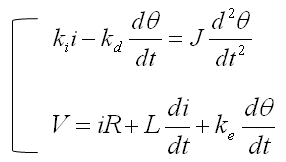DE - Modeling                                                                          Home : www.sharetechnote.com Electro Mechanical System   In this page, we will deal with some examples that are made up of two different domain, electrical domain and mechnical domain. Each of the domain is described by different governing equation. As a result, you will have a system equation that are made up of multiple equations, each of which came from different governing equation.     < Example : DC Motor >   Let's look into one of the simplest electro mechanical system in which a the electrical input (Voltage) is converted to mechnical output (Torque).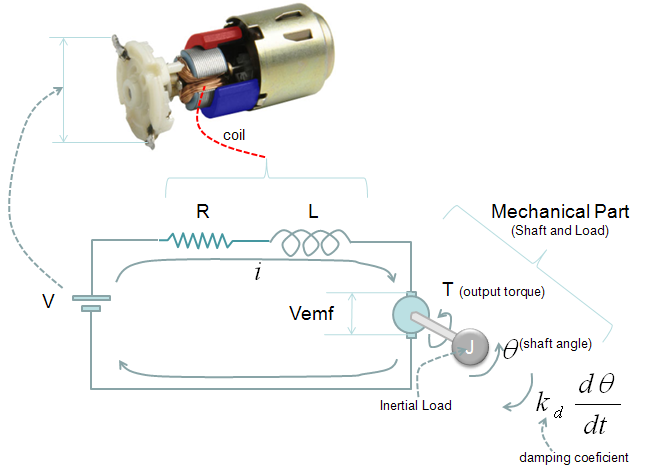The governing equation for the mechanical part for this system can be described as shown below, based on Newton's second law.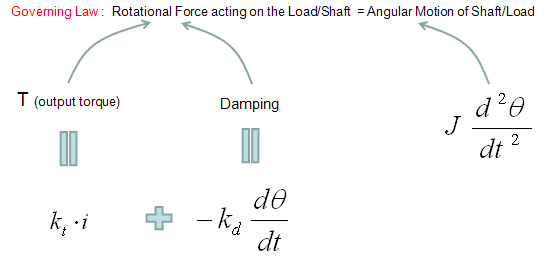From this governing equation, we get a differential equation as shown below. (Some of you may not be familiar with the parameter J. This parameter is called 'Moment Of Inertia'. It is a kind of inertia of an object in rotational movement. The property is very similar to Mass (m) in linear motion. For more formal explanation of this parameter, refer to Moment Of Inertia page)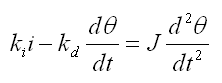Now let's think of the governing equation about the electrical part. It can be described as follows based on Kirchoff's law.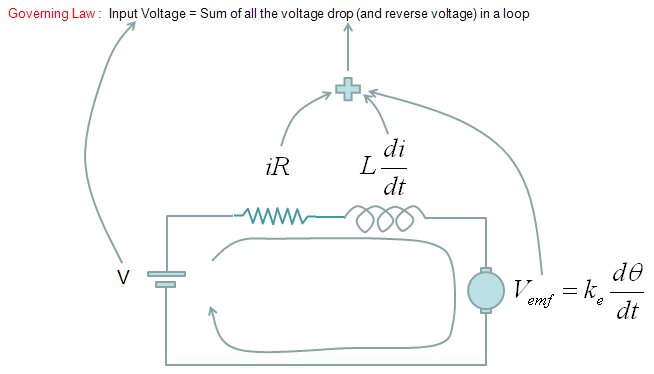From the governing equation shown above and with a little bit of rearrangement, we get the equation as shown below.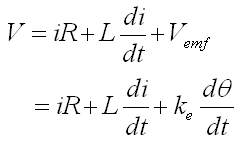By combining the two equations based on two different governing equation, we can get a system equation as shown below.